# The Boolean Satisfiability Problem vilely censured.

## Here I expose some ultimate techniques to make great tools for logic operations. Apologies: someone hacked the article to avoid sharing this technology.

The Boolean Satisfiability Problem (SAT) is the problem of determining if there exists an interpretation that satisfies a given Boolean formula. Considering that a Boolean variable is a variable that can only take two possible values (0 or 1, False or True), a Boolean formula is any operation made with Boolean variables which will be evaluated to take one of the two possible values. That is the why a Boolean value is defined in that way.

So the question is easy: if I give you a formula i.e. “f(x, y, z) = x and (y or z)”, considering and and or Boolean operators, there will be an assignment of (x, y, z ) → (Boolean, Boolean, Boolean) such that f(x, y ,z) = True?

In this post I will show you how to find those values efficiently.

# Some initial operators

In order to ensure that we are able to satisfy any formula first we need to have the necessary operators to represent it. For that purpose, we can use 0 and 1 values to represent False and True values respectively. As it is explained by George Boole in 1847, we can use three intuitive operators where:

• x and y = min(x, y)
• x or y = max(x, y)
• not x = 1- x

But in this post I will use a new operator to replace the or and not. I call it: choice, and when you type (x | y | z) = 1 means you can choose which x, y or z is 1, but only one and exactly one. In fact, you could type (x | y) = 1, which means x = 1 - y = not y.

Considering the · operator A·B = A and B, now we are prepared to show beautiful formulas…

And…, [(x1 | … | xn) = 1] [x1 + … + xn = 1]. Later, we will use another notation, but now let’s study what can we do with this operator.

Proposition. Having the next equation (A|B|C)·(B|D|E)·(C|E|F)=1, where A, B, …, F are Boolean variables, the following statements are fulfilled:

• B = not A and not D
• F = A D

So we can construct the next theorem: for every x, y Boolean values:

• (not x | x and y | z1)·(x and y | not y | z2)·(z1 | z2 | xy) = 1

So, as corollary: we can represent every Boolean formula as a conjunction (·) of choices of Boolean variables.

You can consider some other studies about how different problems (interesting for the Computer Science) could be represented.

# Improving our notation

As mentioned above, each Boolean formula can be expressed as a choice product. Every factor will be called clause: a formula is a product of clauses.

To satisfy a formula we only have to study the relationship between clauses. In this post, the technique that will be used is to face each clause two to two. So, let’s begin with only two clauses: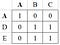(A | B | C)·(A | D | E) = 1

As you can see above, the equation (A | B | C)·(A | D | E) = 1 has only 5 solutions (cases). In fact, we could study the final form of the matrix only by knowing which variables are repeated in each clause. For example,

That is the why we can guess how to combine two matrices which represent different repetitions:

In Python, we can use the numpy library perfectly for this purpose.

`>>> from numpy import matrix>>> Amatrix([[ True, False, False],        [False,  True,  True],        [False,  True,  True]])>>> Bmatrix([[ True, False,  True],        [False,  True, False],        [ True, False,  True]])>>> A&Bmatrix([[ True, False, False],        [False,  True, False],        [False, False,  True]])`

Moreover, we can even implement the matrix that relates any two clauses.

`def cMatrix(clauseC, clauseR):    R = matrix([[True] * len(clauseC)] * len(clauseR))    for i, X in enumerate(clauseC):        if X in clauseR:            j = clauseR.index(X)            R &= matrix([[(i==col) == (j==row) \                         for col in range(len(clauseC))] \                        for row in range(len(clauseR))])    return R`

Now we can test the code considering clauseC is positioned before clauseR in the formula, to order in columns or rows:

`>>> cMatrix((1, 2, 4, 5), (1, 2, 3, 6, 7))matrix([[ True, False, False, False],        [False,  True, False, False],        [False, False,  True,  True],        [False, False,  True,  True],        [False, False,  True,  True]])`

As you can see, we can use numbers or string to represent the Boolean variables. And, one of the most important things: the order of every clause in the formula, as we will study it later.

# Coherence between more than two clauses

We said there is needed more than two clauses to represent any Boolean formula. But, initially we need to ensure us every matrix will face in the correct order every relation.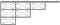Example of a formula of 4 clauses: (A1| … |An)·(B1| … |Bn)·(C1| … |Cn)·(D1| … |Dn) = 1

In the table above, we see we need to represent every relation between every clause in the formula. So if we connect clause-i with clause-j we ensure the clause of the columns is min(i, j) and rows is max(i, j).

Now, I propose the next notation (in my point of view, this will make it all simpler):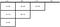Other way to represent (A1| … |An)·(B1| … |Bn)·(C1| … |Cn)·(D1| … |Dn) = 1

Now you can see the 6 = (4·(4–1))/2 faces and other way to represent their corresponding matrices. The values now they represent is the only relation between two clauses, so we are interested in a complete relation [Ai·Bj·Ck·Dl].

So, as you had a katana, you had a stone and you were pretending to sharp the edge cleaning the impurities. You had a way to improve a matrix adding the information of other clause:

In the example we see the next operations:

• [AiCkDl] = ([C1·Dl]·[Ai·C1] |…|[Cn·Dl]·[Ai·Cn])

You can see that we are multiplying a row in matrix(C, D) with a column in matrix(A, C), so we are speaking about a matrix product. The result of that product must take an and operation with the original matrix(A, D).

So,

• For A<C<D: matrix’(A, D) = matrix(A, D) & (matrix(C, D)· matrix(A, C))

Considering a spetial property of those matrices, where the transposed matrix A* is the inverse one (in numpy library is `.transposed()`method in the matrix class):

• A*·A = I (Identity matrix)
• matrix(X, Y)* = matrix(Y, X)

That points the rest of operations:

• For A<C<D: matrix’(C, D) = matrix(C, D) & (matrix(A, D) · matrix(C, A))
• For A<C<D: matrix’(A, C) = matrix(A, C) & (matrix(D, C) · matrix(A, D))

If you configured correctly a class for tables with the matrix class, you could type this code, in instance:

`tables[(C,D)] &= tables[(A, D)]*tables[(C, A)]`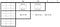You have to use every two matrices before eliminating a clause in the group of matrices

I have an old version of this code not with numpy, and using the inverse order of the structures of tables…, in the file altEng.py and some other explanations from the original article of 2015. If it were required by the users (if this post is of your interest), I could code the entire version in numpy.

If you want to know anymore about the story of this structure I explained this in:

After calculating a matrix with the information of all the clauses, you will notice that you will be able to know if there is or not a solution: the last matrix will have a cell with a True value, indicating that there is at least one case.

Moreover, you can polish the structure to ensure all parts of the edge is sharp; or even sharp the entire structure to get all the cases without any impurities.

For example, after polishing the entire structure this could be a result: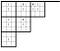Every cell without a 1 is a 0, but a 0 is remarked

Each 1 means that you can reach a solution making True the variables of their corresponded two clauses. So if you wanted to know a solution, you only need a complete sharp matrix and to reconstruct all the formula without two clauses.

That means that you’ll need to add a level of complexity to the algorithm of finding the solution.

Perhaps, with a polished structure you could guess faster a solution. Well, that is the reason of this section: in the figure above you can find exactly three solutions. Let’s see that better with the next figure:

The three cases are entangled in faces where we only can know if there is a case or not in each cell. The two Blue cases have in common with the two Orange cases the Purple case.

We know there is a superposition because there is a triplet with two ones and a zero, as was exposed in the first figure. So, if you want to collapse to observe exclusive cases, you only have to decide what of those 1’s must be converted in 0…, then you’ll polish and you will find at least one case.

After eliminating the entanglements, you will see trivial assignments to complete the solutions. Only eliminating cases you can reach the solutions without reconstructing the complete formula.

In my old code I implemented a way to index cases without corroborating entanglements (typing `object[case]`). For that reason the solution is fulfilled fastly or not fulfilled for that index. Considering that it is able to find a solution in most indices, as it usually works with a high probability (80%) then you can always try another index to find the desired solution.

Based in this result I considered my demonstration that SAT is solvable in a polynomial time and space.

Time and users will tell us if those techniques are exactly what I ensure.